# uexGetui查看3.0文档

#### 1、 简介

###### 1.2 使用插件需要的相关配置

• 替换第 12 行到 23 行中 "你的APPID"、"你的APPKEY"、"你的APPSECRET" 为申请到的对应值。
``````<meta-data
android:name="PUSH_APPID"
android:value="你的APPID" />
<meta-data
android:name="PUSH_APPKEY"
android:value="你的APPKEY" />
<meta-data
android:name="PUSH_APPSECRET"
android:value="你的APPSECRET" />
<meta-data
android:name="PUSH_GROUPID"
android:value="" />
``````
• 适配 Android 5.0 以上需要修改自定义权限名称，替换第 4 行到 8 行 name 值中 "第三方包名" 为自己的包名。

``````<uses-permission android:name="getui.permission.GetuiService.第三方包名" />
<permission
android:name="getui.permission.GetuiService.第三方包名"
android:protectionLevel="normal" >
</permission>
``````

#### 2、API概览

###### changePushStatus //开始或暂停推送服务

• status: (Int 类型) 必选 0 代表开始推送，1 代表暂停推送。

• Android 2.2+

• 3.0.0+

• Android
``````uexGetui.changePushStatus(0);
``````
###### destroy //停止并销毁推送服务

• Android 2.2+
• iOS 6.0+

• 3.0.0+

``````uexGetui.destroy();
``````
###### version //获取SDK版本号

• Android 2.2+
• iOS 6.0+

• 3.0.0+

``````uexGetui.version();
uexGetui.onGetVersion = function(opId, dataType, data){
// Handle data
}
``````
###### initialize //初始化推送服务

• Android

• iOS
• appId: 申请到的 appId
• appKey: 申请到的 appKey
• appSecret: 申请到的 appSecret

• Android 2.2+
• iOS 6.0+

• 3.0.0+

• Android
``````uexGetui.initialize();
uexGetui.onInitializePusher = function(opId, dataType, data){
// Handle data
}
``````
• iOS
``````var params = {
appId:'h5AH1rK4KW7vfFGZjT7fG',
appKey:'aiMe49ehZa7IK8sevOTiY8',
appSecret:'wx7779c7c063a9d4d9'
};
var data = JSON.stringify(params);
uexGetui.initialize(data);
uexGetui.onInitializePusher = function(opId, dataType, data){
// Handle data
}
``````
###### clientId //获取当前用户的clientId

• Android 2.2+
• iOS 6.0+

• 3.0.0+

``````uexGetui.clientId();
uexGetui.onGetClientId = function(opId, dataType, data){
// Handle data
}
``````
###### setTags //为用户设置标签

• tags: (String 类型) 必选 标签字符串（以逗号,分割）
• sn:用户自定义的序列号,用来唯一标识该动作, 自定义 receiver 中会回执该结果

• Android 2.2+
• iOS 6.0+

• 3.0.0+

``````var params = "tag1,tag2";
var sn="getui";
uexGetui.setTags(params,sn);
uexGetui.onSetTags = function(opId, dataType, data){
// Handle data
}
``````
###### bindAlias //绑定用户别名

• alias: (String 类型) 必选 别名字符串

• Android 2.2+
• iOS 6.0+

• 3.0.0+

``````var alias = "name";
var isSelf="true";
uexGetui.bindAlias(alias,isSelf);
uexGetui.onBindAlias = function(opId, dataType, data){
// Handle data
}
``````
###### unbindAlias //解绑用户别名

• alias: (String 类型) 必选 别名字符串
• isSelf:(boolean类型)必选，是否只对当前 cid 有效,如果是 true,只对当前cid做解绑;如果是 false,对所有绑定该别名的 cid列表做解绑 平台支持

• Android 2.2+

• iOS 6.0+

• 3.0.0+

``````var alias = "name";
var isSelf="true";
uexGetui.unbindAlias(alias,isSelft);
uexGetui.onUnbindAlias = function(opId, dataType, data){
// Handle data
}
``````
###### getPushStatus //获取推送服务的服务状态

• Android 2.2+
• iOS 6.0+

• 3.0.0+

``````uexGetui.getPushStatus();
uexGetui.onGetPushStatus = function(opId, dataType, data){
// Handle data
}
``````
###### sendMessage //发送上行消息到应用服务器

• taskId: (String 类型) 必选 task id
• data: (String 类型) 必选 需要发送的字符串内容

• Android 2.2+
• iOS 6.0+

• 3.0.0+

``````var params = {
data:'any string'
};
var data = JSON.stringify(params);
uexGetui.sendMessage(data);
uexGetui.onSendMessage = function(opId, dataType, data){
// Handle data
}
``````
###### sendFeedbackMessage //上行第三方自定义回执

• taskId: (String 类型) 必选 task id
• messageId: (String 类型) 必选 message id
• actionId: (String 类型) 必选 action id

• Android 2.2+

• 3.0.0+

• Android
``````var params = {
messageId:'MESSAGE_ID',
actionId:90001
};
var data = JSON.stringify(params);
uexGetui.sendFeedbackMessage(data);
uexGetui.onSendFeedbackMessage = function(opId, dataType, data){
// Handle data
}
``````
###### setSilentTime //设置静默时间

• beginHour: (Int 类型) 必选 开始时间 0-23
• duration: (Int 类型) 必选 持续时间 0-23

• Android 2.2+

• 3.0.0+

• Android
``````var params = {
beginHour:10,
duration:6
};
var data = JSON.stringify(params);
uexGetui.setSilentTime(data);
uexGetui.onSetSilentTime = function(opId, dataType, data){
// Handle data
}
``````
###### registerDeviceToken //注册设备token

• iOS 6.0+

• 3.0.0+

``````uexGetui.registerDeviceToken();
uexGetui.onRegisterDeviceToken = function(opId, dataType, data){
// Handle data
}
``````
###### registerVoipToken //注册VOIPtoken

• iOS 8.0+

• 3.0.0+

``````uexGetui. registerVoipToken();
uexGetui. onRegisterVoipToken = function(opId, dataType, data){
// Handle data
}
``````
###### onGetVersion //取得SDK 版本号监听方法

• opId:(Int 类型) 操作ID
• dataType:(Int 类型) 数据类型 详见CONSTANT中Callback方法数据类型
• data:(String 类型) 版本字符串

• Android 2.2+
• iOS 6.0+

• 3.0.0+

``````uexGetui.onGetVersion = function(opId, dataType, data){
}
``````
###### onInitializePusher //初始化推送服务监听方法

• opId:(Int 类型) 操作ID
• dataType:(Int 类型) 数据类型 详见CONSTANT中Callback方法数据类型
• data:(String 类型) JSON 字符串
• result 初始化结果：成功 0，失败 1。
• clientId 注册的 ClientId
• error 错误消息（初始化成功错误消息为空）

• Android 2.2+
• iOS 6.0+

• 3.0.0+

``````uexGetui.onInitializePusher = function(opId, dataType, data){
var object = JSON.parse(data);
var result = object.result;
var clientId = object.clientId;
var error = object.error;
}
``````
###### onSetTags //设置用户标签监听方法

• opId:(Int 类型) 操作ID
• dataType:(Int 类型) 数据类型 详见CONSTANT中Callback方法数据类型
• data:(Int 类型) 设置用户标签结果

• Android 2.2+
• iOS 6.0+

• 3.0.0+

``````uexGetui.onSetTags = function(opId, dataType, data){
}
``````
###### onGetPushStatus //获取推送服务的服务状态监听方法

• opId:(Int 类型) 操作ID
• dataType:(Int 类型) 数据类型 详见CONSTANT中Callback方法数据类型
• data:(Int 类型) 推送服务状态

• Android 2.2+
• iOS 6.0+

• 3.0.0+

``````uexGetui.onGetPushStatus = function(opId, dataType, data){
}
``````
###### onGetClientId //获取当前用户的clientId监听方法

• opId:(Int 类型) 操作ID
• dataType:(Int 类型) 数据类型 详见CONSTANT中Callback方法数据类型
• data:(String 类型) ClientId 字符串

• Android 2.2+
• iOS 6.0+

• 3.0.0+

``````uexGetui.onGetClientId = function(opId, dataType, data){
}
``````
###### onBindAlias //绑定用户别名监听方法

• opId:(Int 类型) 操作ID
• dataType:(Int 类型) 数据类型 详见CONSTANT中Callback方法数据类型
• data:(Int 类型) 绑定别名结果

• Android 2.2+
• iOS 6.0+

• 3.0.0+

示例代码

``````uexGetui.onBindAlias = function(opId, dataType, data){
}
``````
###### onUnbindAlias //解绑用户别名监听方法

• opId:(Int 类型) 操作ID
• dataType:(Int 类型) 数据类型 详见CONSTANT中Callback方法数据类型
• data:(Int 类型) 解绑别名结果

• Android 2.2+
• iOS 6.0+

• 3.0.0+

``````uexGetui.onUnbindAlias = function(opId, dataType, data){
}
``````
###### onSendMessage //发送上行消息监听方法

• opId:(Int 类型) 操作ID
• dataType:(Int 类型) 数据类型 详见CONSTANT中Callback方法数据类型
• data:(Int 类型) 发送上行消息结果

• Android 2.2+
• iOS 6.0+

• 3.0.0+

``````uexGetui.onSendMessage = function(opId, dataType, data){
}
``````

• opId:(Int 类型) 操作ID
• dataType:(Int 类型) 数据类型 详见CONSTANT中Callback方法数据类型
• data:(String 类型) JSON 消息字符串
• result 注册结果：成功 0，失败 1。
• appId 应用 Id
• data 消息内容

• Android 2.2+
• iOS 6.0+

• 3.0.0+

``````uexGetui.onReceiveMessage = function(opId, dataType, data){
var object = JSON.parse(data);
var result = object.result;
var appId = object.appId;
var message = object.data;
}
``````
###### onRegisterDeviceToken //注册设备token监听方法

• opId:(Int 类型) 操作ID
• dataType:(Int 类型) 数据类型 详见CONSTANT中Callback方法数据类型
• data:(String 类型) JSON 消息字符串
• result 注册结果：成功 0，失败 1。
• token 获得的 token 字符串
• error 错误消息（成功时错误消息为空）

• iOS 6.0+

• 3.0.0+

``````uexGetui.onRegisterDeviceToken = function(opId, dataType, data){
var object = JSON.parse(data);
var result = object.result;
var token = object.token;
var error = object.error;
}
``````
###### onRegisterVoipToken //注册VOIPtoken监听方法

• opId:(Int 类型) 操作ID
• dataType:(Int 类型) 数据类型 详见CONSTANT中Callback方法数据类型
• data:(String 类型) JSON 消息字符串
• result 注册结果：成功 0，失败 1。
• token 获得的VOIP token 字符串
• error 错误消息（成功时错误消息为空）

• iOS 8.0+

• 3.0.0+

``````uexGetui. onRegisterVoipToken = function(opId, dataType, data){
var object = JSON.parse(data);
var result = object.result;
var token = object.token;
var error = object.error;
}
``````
###### onErrorOccur //错误发生监听方法

• opId:(Int 类型) 操作ID
• dataType:(Int 类型) 数据类型 详见CONSTANT中Callback方法数据类型
• data:(String 类型) 错误消息字符串

• iOS 6.0+

• 3.0.0+

``````uexGetui.onErrorOccur = function(opId, dataType, data){
}
``````
###### onSetSilentTime //设置静默时间监听方法

• opId:(Int 类型) 操作ID
• dataType:(Int 类型) 数据类型 详见CONSTANT中Callback方法数据类型
• data:(Int 类型) 设置静默结果

• Android 2.2+

• 3.0.0+

``````uexGetui.onSetSilentTime = function(opId, dataType, data){
}
``````
###### onSendFeedbackMessage //上行第三方自定义回执监听方法

• opId:(Int 类型) 操作ID
• dataType:(Int 类型) 数据类型 详见CONSTANT中Callback方法数据类型
• data:(Int 类型) 上行第三方自定义回执结果

• Android 2.2+

• 3.0.0+

``````uexGetui.onSendFeedbackMessage = function(opId, dataType, data){
}
``````

• Android 2.2+

• 3.0.0+

``````uexGetui.onNotificationArrived = function(opId, dataType, data){
}
``````

• Android 2.2+

• 3.0.0+

``````uexGetui.onNotificationClicked = function(opId, dataType, data){
}
``````

#### 3、更新历史

iOS 更新功能： API 版本：uexGetui-2.3.0.0(iOS)

• 最近更新时间：`2018-07-26`

2.3.0.0 新增 AppLink 服务及统计支持。

1.6.2.0 添加 HTTPDNS 域名解析，防止域名劫持。

*添加 UserNotifications.framework 使用，以 Optional 弱引用方式引用
1.0.0 个推 SDK

Android更新功能： API 版本： uexGetui-2.10.3.5（Android）

• 最近更新时间：`2017-08-11`

bug修复
2.10.2.0 消息和透传的接收方式由广播改为 IntentService，避免小概率的广播丢失。

1.0.0 个推 SDK

# 个推开发者平台创建应用

#### 4、打开 个推开发者平台注册成为开发者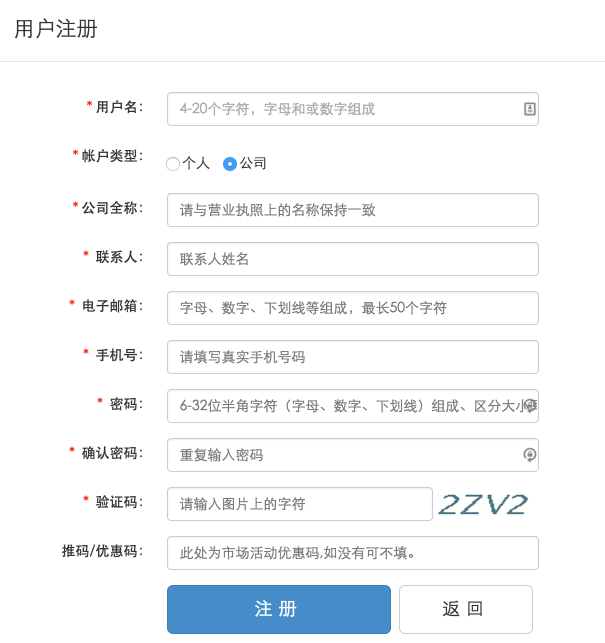#### 5、 注册成功后登陆并登记应用

Android 应用标识填写自定义的包名。 iOS 应用证书选择导出的 p12 格式推送证书，密码为导出时创建的密码。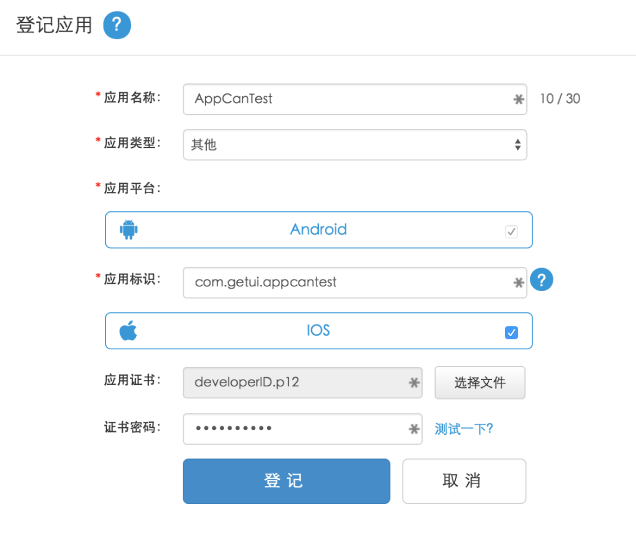#### 7、在线打包

###### 7.1 ×选择上传到打包服务器的自定义插件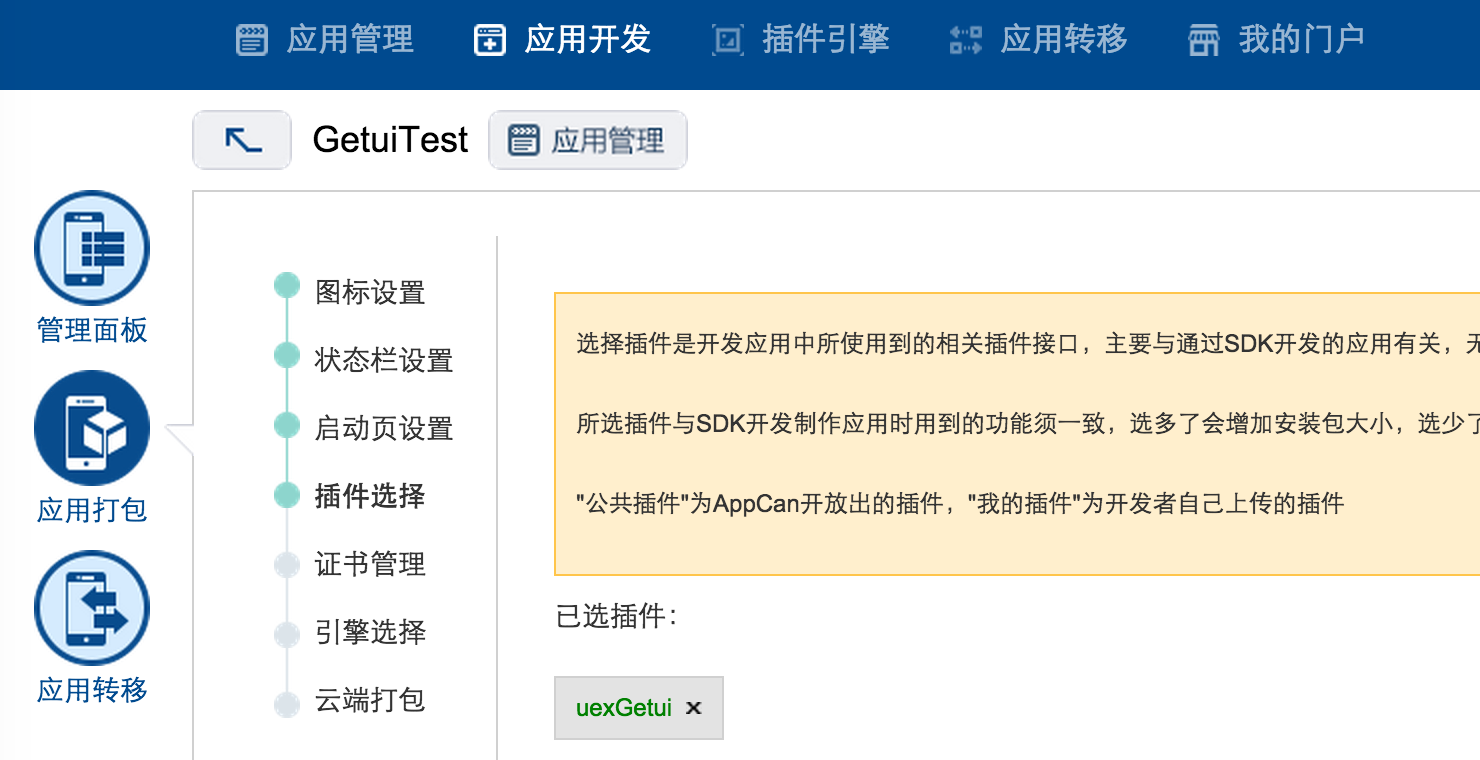###### 7.2 ×选择证书填写自定义包名进行打包（安卓）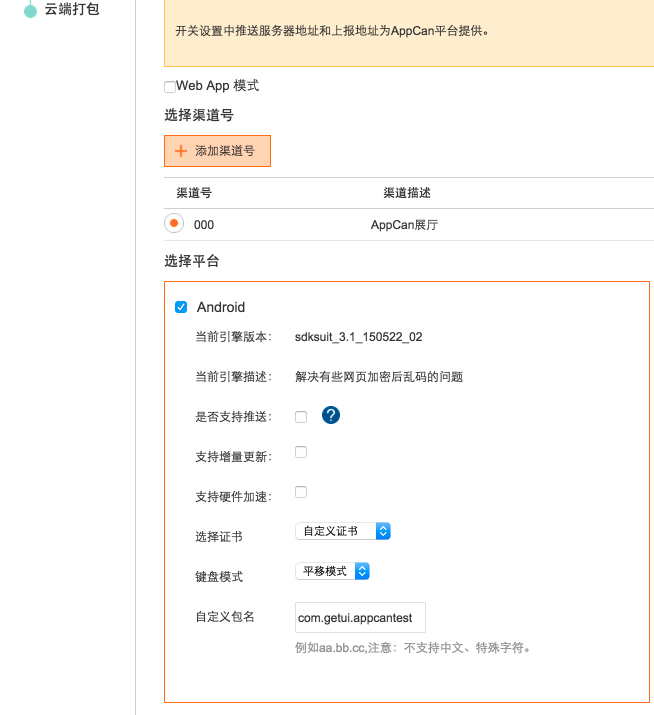###### 7.3 × ios应用需要上传申请的苹果证书，打包选择对应的证书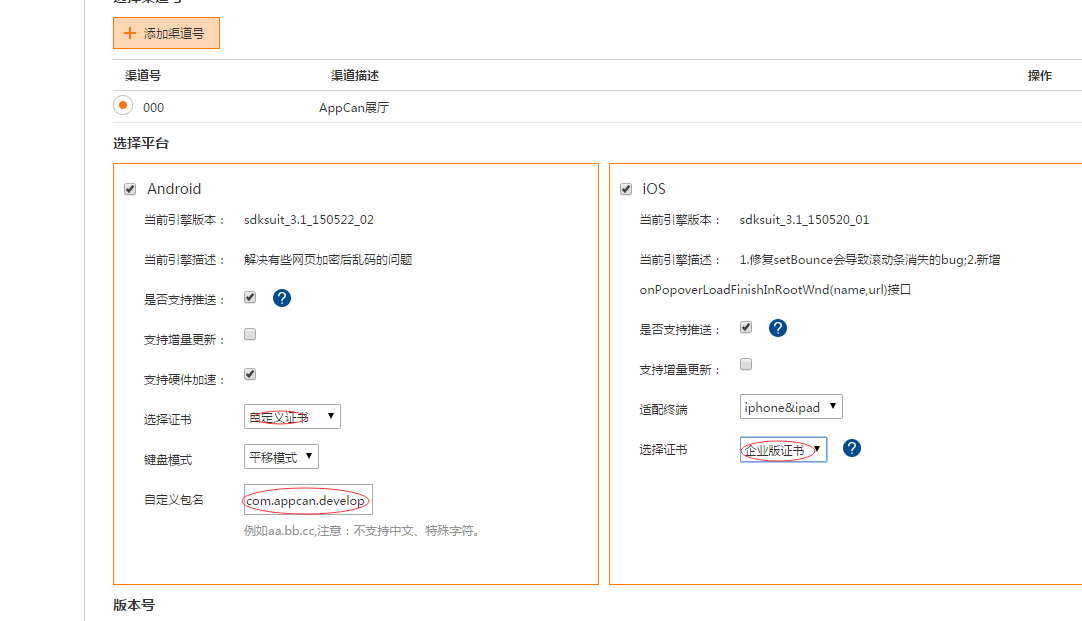最后， 跳转到个推开发者平台，创建推送